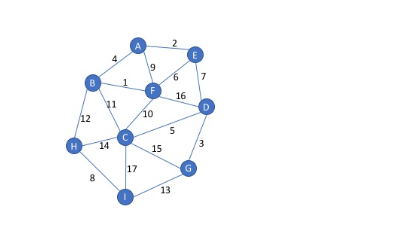# Quiz 11 – Graphs Solved

25.00 \$

Category:

## Description

Dijkstra’s algorithm finds the shortest path

from one specified vertex to all the other vertices

from one specified vertex to another specified vertex

from all vertices to all other vertices that can be reached along one edge

from all vertices to all other vertices that can be reached along multiple edges

Question 2
/ 2 pts
In a weighted graph, the minimum spanning tree tries to minimize

The number of edges from the starting vertex to a specified vertex

The number of edges connecting all the vertices

the total weight of the edges fro the starting vertex to a specified vertex

the total weight of edges connecting all the vertices

Question 3
/ 6 pts

What is the order that edges are added when using Prim’s algorithm to find the minimum spanning tree for this graph.  Start with vertex A.  Type edges as two letters with spaces between them, e.g. AB BC CD